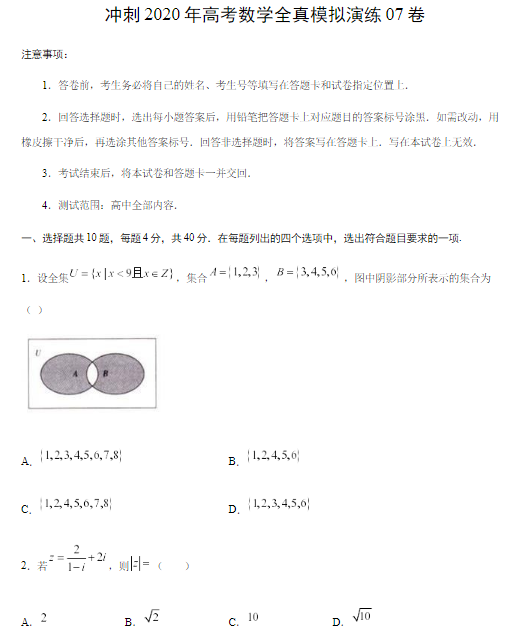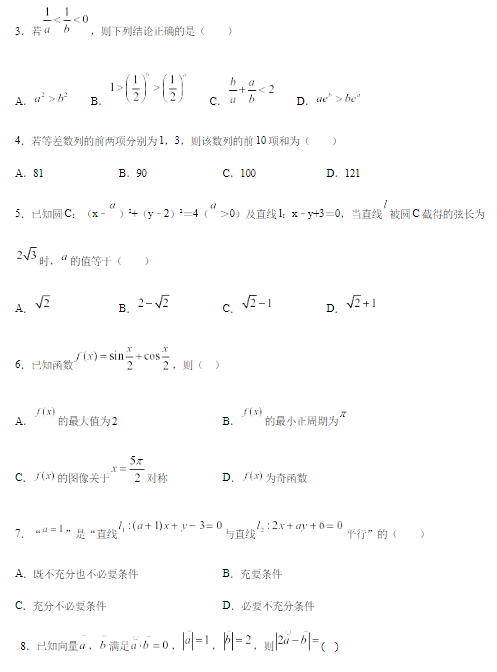# 必刷题电子版哪里可以下载？北京考生们来这里找了

2020-08-05 20:39:11 　来源：网络整理点击领取>>> 《北京全9科高考必刷题及答案》pdf高清版选择题速解小窍门

1

排除法、代入法

当从正面解答不能很快得出答案或者确定答案是否正确时，可以通过排除法，排除其他选项，得到正确答案。排除法可以与代入法相互结合，将4个选项的答案，逐一带入到题目中验证答案。

例题：2014年高考全国卷Ⅰ理数第11题已知函数f(x)=ax3-3x2+1,若f(x)存在唯一的零点x0,且x0>0，则a的取值范围为：

A、(2，+∞) B、(-∞，-2) C、(1，+∞) D、(-∞，-1)

解析：取a=3,f(x)=3x3-3x2+1，不合题意，可以排除A与C;取a=-4/3,f(x)=-4x3/3-3x2+1，不合题意，可以排除D;故只能选B

2

特例法

有些选择题涉及的数学问题具有一般性，这类选择题要严格推证比较困难，此时不妨从一般性问题转化到特殊性问题上来，通过取适合条件的特殊值、特殊图形、特殊位置等进行分析，往往能简缩思维过程、降低难度而迅速得解。

例题：2016年高考全国卷Ⅱ理数第12题

已知函数f(x)(x∈R)满足f(-x)=2-f(x),若函数y=x+1/x与y=f(x)图像焦点为为(x1,y1),(x2,y2),…，(xm,ym),则∑mi=1(xi+yi)=( )

A、0 B、m C、2m D、4m

解析：由f(-x)=2-f(x)得，f(x)关于(0,1)对称，故可取符合题意的特殊函数f(x)=x+1,联立y=x+1,y=x+1/x,解得交点为(-1,0)和(1,2)，所以∑2i=1(xi+yi)=(x1+y1)+(x2+y2)=(-1+0)+(1+2)=2，此m=2，只有选项B符合题意。

3

极限法

当一个变量无限接近一个定量，则变量可看作此定量。对于某些选择题，若能恰当运用极限法，则往往可使过程简单明快。

例题：对任意θ∈(0，π/2)都有( )

A sin(sinθ)

B sin(sinθ)>cosθ>cos(cosθ)

C sin(cosθ)

D sin(cosθ)

解析：当θ→0时，sin(sinθ)→0，cosθ→1，cos(cosθ)→cos1，故排除A与B;当θ→π/2时，cos(sinθ)→cos1，cosθ→0，故排除C，只能选D。QQ扫一扫您将获得群内福利家长互动海量资料同步免费视频课程了解最新信息及时解读资讯你可能感兴趣的文章立即咨询1对1在线课程*学而思爱智康会在24小时内与您取得电话联系大家都在看热门课程介绍限时免费领取学习相关

• 全国课程在线咨询
• 咨询热线：4000-121-121
• 扫描注册有礼

• 让学习更有效